# Effect of Source Inductance on Single Phase Converter

We know that in converters the commutation of thyristors takes place instantaneously once there is a phase reversal. But in practice, there exists an inductance and resistance in the supply source. This inductance is known as source inductance LS and due to this the line commutation process of the thyristors and the turning ON process of the thyristors are delayed for a small duration, which is termed as the Overlapping Period.

In order words, due to the presence of source inductance, the current in the outgoing thyristor takes a finite time to reduce to zero. This causes a delay in the commutation of that thyristor and at the same time, the current will rise in the incoming thyristor. This results in the conduction of both incoming and outgoing thyristor simultaneously for a finite time period known as the Overlapping Period.

During the overlapping period, the rate of rise of load current through the thyristors being turned ON will be equal to the rate of fall of current in the thyristors being turned OFF, this results in the short circuit across the source terminals. Hence, the output voltage becomes zero during the overlap period and this period remains for a duration μ called as overlap angle or commutation angle.

The overlapping period in converters due to source inductance affects the performance characteristics of the converter, it reduces the average output voltage and displacement factors. Let us see the effect of source inductance on a single-phase converter.

## Effect of Source Inductance on Single Phase Converter :

The phenomenon of the overlapping period is more severe in fully-controlled converters compared to the semi-converters. The below shows the single-phase fully-controlled bridge converter circuit with source inductance LS.

During the positive half of the supply cycle, thyristors T1 and T2 are triggered at firing angle α. The positive cycle will reverse bias the already conducting SCRs T3 and T4. The positive group SCRs T1 and T2 now act as incoming SCRs whereas the negative group SCRs T3 and T4 will act as outgoing SCRs.

Since the load current is assumed to be constant, it should immediately transfer from outgoing SCRs to incoming SCRs, i.e., from T3 T4 to T1 T2. The current, i2 due to T3 and T4 should go to zero instantaneously and the current, i1 due to T1 and T2 should reach the load current Idc instantaneously.

We know that an inductor does not allow sudden changes in current. Thus due to source inductance LS, the fall of i2 and rise of i1 will not be instantaneous rather it is gradual. Hence, there will be a small period during which both the currents i1 and i2 will flow in the circuit, resulting in a short circuit across the source terminals. This period is called as Overlapping Period.

During this period, the output voltage will be zero. The above shows the typical waveform of voltage and current of the converter with source inductance LS. At ωt = π, as the supply voltage is negative the thyristors T1 and T2 should be turned off, and hence i1 should be zero.

But due to the presence of source inductance, the current i1 will not go to zero at ωt = π, rather starts decreasing gradually towards zero. Due to this, the output voltage Vdc will become negative for a small period till the incoming SCRs are triggered.

## Derivation of Output Voltage :

#### Below shows the equivalent circuit for a the above bridge converter circuit.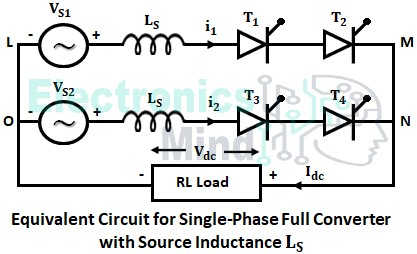Applying KVL to the loop LMNOL, (adsbygoogle = window.adsbygoogle || []).push({});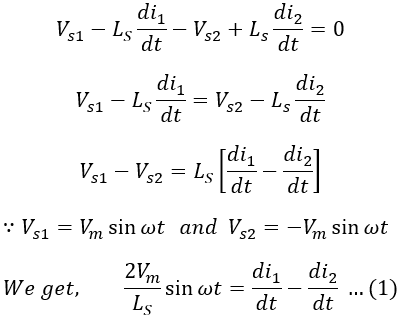Since the load current is constant,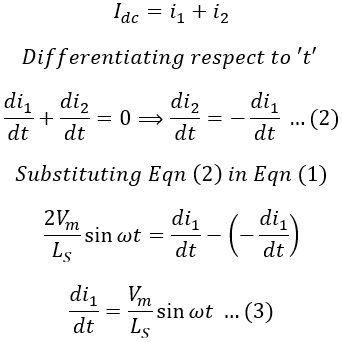At the instant ωt = α, load current i1 through T1 and T2 builds up from 0 to Idc during the overlap angle μ.i1 = 0, at ωt = (α + μ)i1 = IdcIntegrating equation (3) we get, (adsbygoogle = window.adsbygoogle || []).push({});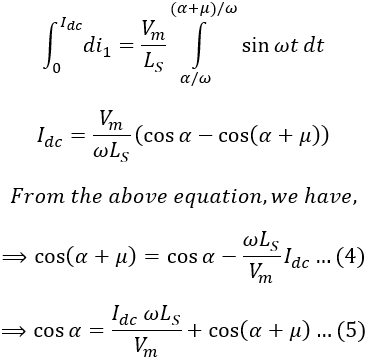From the waveform, we have,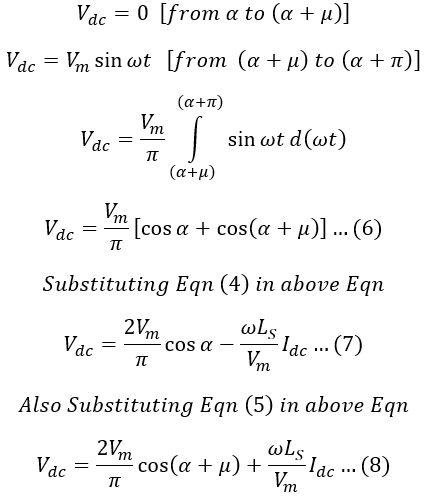By using equation (7), a dc equivalent circuit for a single-phase full converter is given as,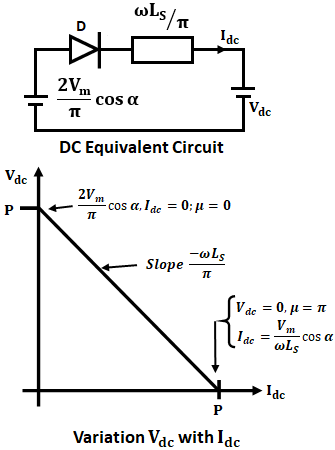This reveals the effect of source inductance LS is to present an equivalent inductance ωLS/π which is in series with internal voltage (ωVm cos α)/π. If Idc is the load current, the presents of source inductance cause a drop of (ωLS Idc)/π in the load or output voltage. From the above variation curve of load voltage with load current, we can conclude that the average output voltage decreases with the increase in source inductance.

Therefore, the effect of source inductance on a single-phase full converter is such that, until the overlap angle lies below π, the output voltage will be equal to a value shown in equation (6). If μ = π, both incoming and outgoing thyristors will remain in the conduction state, thereby making the output voltage zero.